# The Paper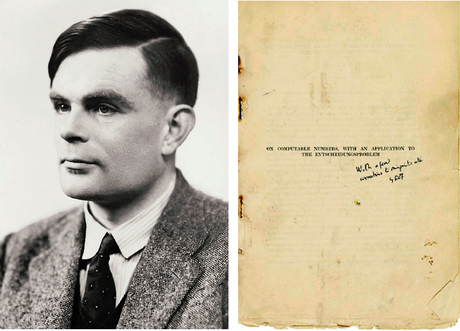Author: Alan Turing

Published: Journal of Math, Volume 58, 1936

TL;DR: Turing invents a programmable machine to show the power of computation and to expose the limits of formal mathematical systems.

Image Source

# Computable Numbers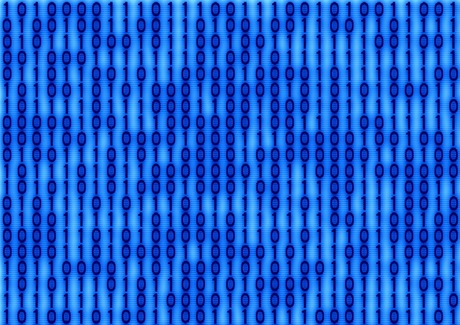“The real numbers whose expressions as a decimal are calculable by finite means. ”

“A number is computable if its decimal can be written down by a machine.”

Turing’s computable numbers will be binary numbers in the range 0 to 1.

For example, 1/3 in binary is:

``0.0101010101010101...``

How is 1/3 equal to 0.0101010101010101… in binary?

``````1/3 = 0.0101010101010101...
1/3 = (0 * 2^-1) + (1 * 2^-2) + (0 * 2^-3) + (1 + * 2^-4) + ...
1/3 = 1/4 + 1/16 + 1/64 + 1/256 + ... ``````

Image Source

# EntscheidungsproblemPronounced: Ent-shy-dungs-prob-lem

Entscheidungs is a German word for decision/decidability.

## Hilbert’s Ideal Formal Mathematical System

1. Consistent - No contradictory well-formed formula.
2. Complete - All true formulas can be derived from the axioms.
3. Decidable - Finite decision procedure exists to determine provability of any given well-formed formula.

The Entscheidungsproblem or decision problem involves finding the procedure mentioned in item three of this list.

Image Source

# Mr. Turing Needs a Machine

Heinrich Behmann on the Entscheidungsproblem:

“It is of fundamental importance for the character of this problem that only mechanical calculations according to given instructions, without any thought activity in the stricter sense, are admitted as tools for the proof. One could, if one wanted to, speak of mechanical or machinelike thought.

Perhaps one could later let the procedure be carried out by a machine.

For Turing to show the Entscheidungsproblem unsolvable he first needs to invent such a machine.

# Human Computation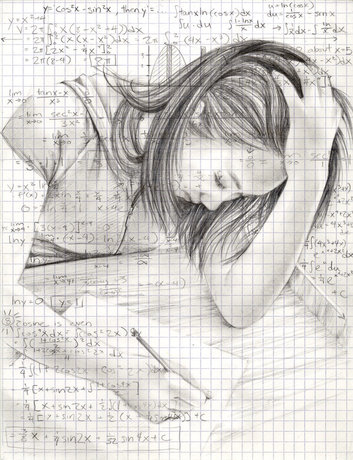Turing first investigates how humans work through calculations.

When performing a computation the human has:

• A finite number of symbols with which to work.
• Discrete states of mind they can switch between.
• A paper on which to perform calculations and record results.

Image Source

# State Machines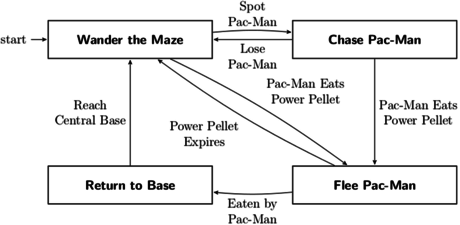An abstract machine that can be in one of a finite number of states.

The machine is in only one state at a time, its current state.

It can transition from one state to another when initiated by a triggering event.

Image Source

# Turnstile State Machine Analogy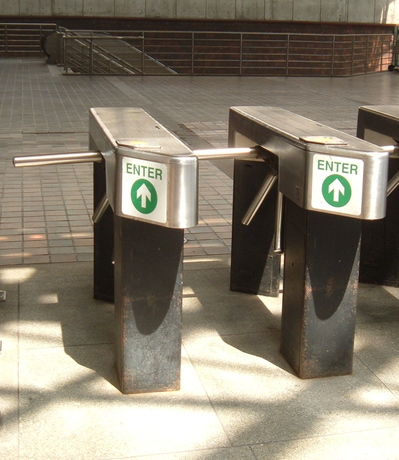A turnstile is a type of one-way gate.

Payment unlocks for a single person.

Image Source

# Turnstile State Machine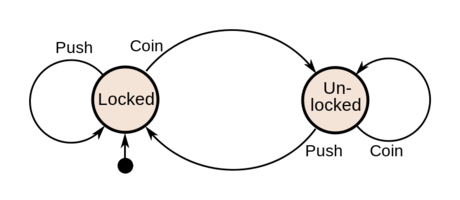A one-way turnstile used for subways modeled as a state machine.

Current State Input Next State Output
Locked coin Unlocked Unlock turnstile so customer can push through.
push Locked None
Unlocked coin Unlocked None
push Locked When customer has pushed through, lock turnstile.

### Resources

Image Source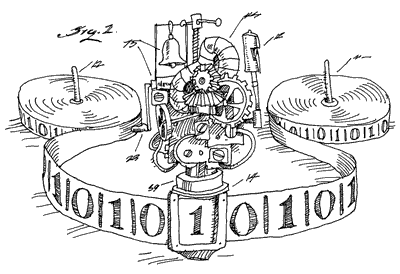Turing’s machines are state machines with the ability to read from and write to a tape.

The tape is divided into squares each capable of bearing a symbol.

The tape is of infinite length.

Image Source

# Turing MachinesState Machine + Tape = Simplest Computer

The machine state combined with the currently scanner symbol is called the configuration.

• A change in the state
• Writing a symbol to a blank square
• Erasing a symbol from a square
• Moving the tape by one square in either direction

Image Source

# Examples of Turing Machine

Turing machine for printing out the binary form of 1/3:

Configuration Behaviour
State Symbol Print Move Next State
a blank Print 0Move R b
b blank Print 1Move R a

# Binary Counter Machine

Turing machine implementing a binary counter:

Configuration Behaviour
State Symbol Print Move Next State
begin blank Print 0 increment
increment blank Print 1 rewind
increment 0 Print 1 rewind
increment 1 Print 0Move L increment
rewind blank Move L increment
rewind 0 Move R rewind
rewind 1 Move R rewind

# Circular or Circle-Free Machines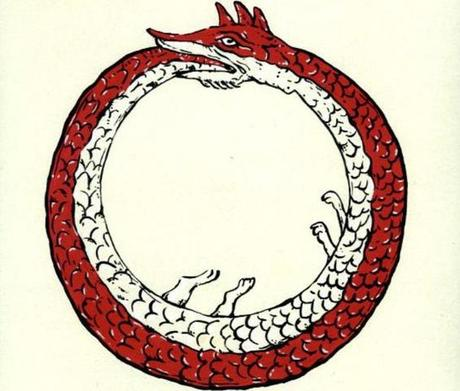Once set in motion a Turing machine will write an infinite number of figures to it’s tape.

A circular machine is one that eventually stops writing figures.

A non-circular machine is said to be circle-free.

* * *

Both types of machines will run for an infinite length of time, the difference is their output.

A circle-free machine produces results forever, while a circular machine will eventually get stuck in a non-productive loop.

Image Source

# Encoding Turing Machines

Turing Machines can be described as quintuples `q s q' s' d` separated by semicolons.

• `q` is the current state
• `s` is the symbol currently under the read/write head
• `q'` is the next state to enter
• `s'` is the symbol to be written in place of `s`
• `d` is the direction the tape head should move

A descriptions of the 1/3 machine could be written as:

a _ b 0 R ; b _ a 1 R

The complete configuration of a machine is its current state and its current tape.

Snapshots of the complete configuration of the 1/3 machine separated by colons:

``a:b0:0a1:01b0:010a1:0101b0:01010a1:...``

The state is recorded directly in front of the current tape read/write position.

# The Universal Turing Machine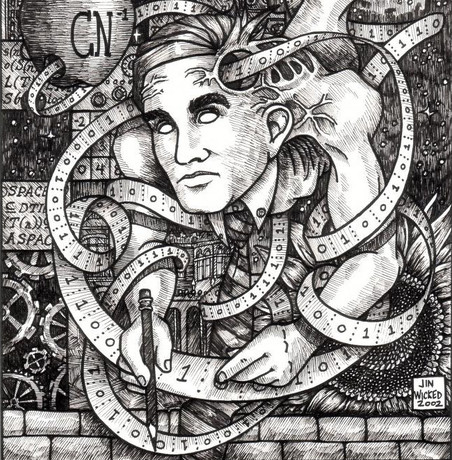Given that we can capture as a string of symbols:

1. Any Turing machine description.
2. The state and tape of a Turing machine at any point in time.

We can build a Turing machine `U` that can take as input from its tape the description of any other Turing machine `M`.

This machine `U` will output successive snapshots of the state and tape of `M`.

In the paper, Turing describes how to build this machine-simulating machine, which he calls a universal machine.

Image Source

# Limits of Computation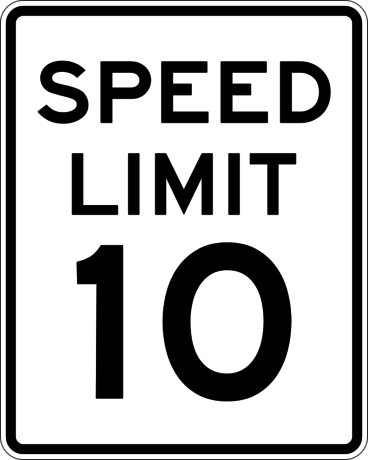## 1. An Uncomputable Number

A number that can be described but not computed.

## 2. An Impossible Machine

The Halting Problem cannot be solved.

## 3. Entscheidungsproblem

A halting problem hides within first order predicate logic.

Demonstration on whiteboard.

### Resources

Image SourceThe New Turing Omnibus - A. K. Dewdney 66 Excursions in Computer Science

The Annotated Turing - Charles Petzold

A Guided Tour through Alan Turing’s Historic Paper on Computability and the Turing Machine

Cryptonomicon - Neal Stephenson

A novel about WWII cryptography and modern-day data havens with Turing as one of the characters.

Gödel, Escher, Bach - Douglas Hofstadter

An Eternal Golden Braid